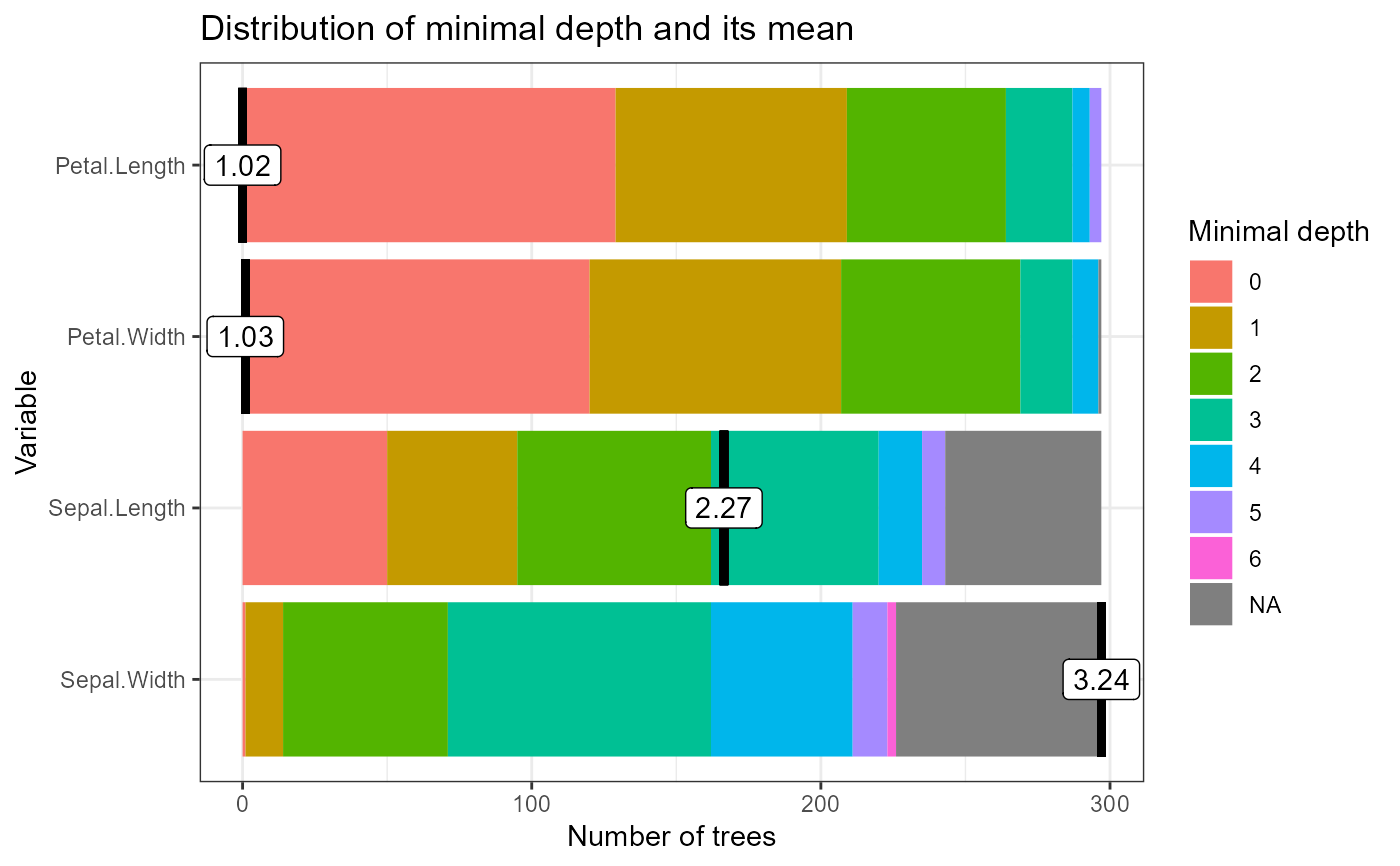Plot the distribution of minimal depth in a random forest

plot_min_depth_distribution(
min_depth_frame,
k = 10,
min_no_of_trees = 0,
mean_sample = "top_trees",
mean_scale = FALSE,
mean_round = 2,
main = "Distribution of minimal depth and its mean"
)

## Arguments

min_depth_frame A data frame output of min_depth_distribution function or a randomForest object The maximal number of variables with lowest mean minimal depth to be used for plotting The minimal number of trees in which a variable has to be used for splitting to be used for plotting The sample of trees on which mean minimal depth is calculated, possible values are "all_trees", "top_trees", "relevant_trees" Logical: should the values of mean minimal depth be rescaled to the interval [0,1]? The number of digits used for displaying mean minimal depth A string to be used as title of the plot

A ggplot object

## Examples

forest <- randomForest::randomForest(Species ~ ., data = iris, ntree = 300)
plot_min_depth_distribution(min_depth_distribution(forest))